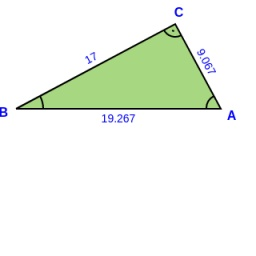# Circumscribed 6568

In a right triangle ABC with a right angle at the vertex C, it is given: a = 17cm, Vc = 8 cm. Calculate the length of the sides b, c, its area S, the perimeter o, the length of the radii of the circles of the triangle circumscribed by R and inscribed r and the magnitude of the angles alpha and beta.

b =  9.0667 cm
c =  19.2667 cm
S =  77.0667 cm2
o =  45.3333 cm
A =  61.9275 °
B =  28.0725 °
r =  3.4 cm
R =  9.6333 cm

### Step-by-step explanation:

Try calculation via our triangle calculator.Did you find an error or inaccuracy? Feel free to write us. Thank you!

Tips for related online calculators
Looking for a statistical calculator?